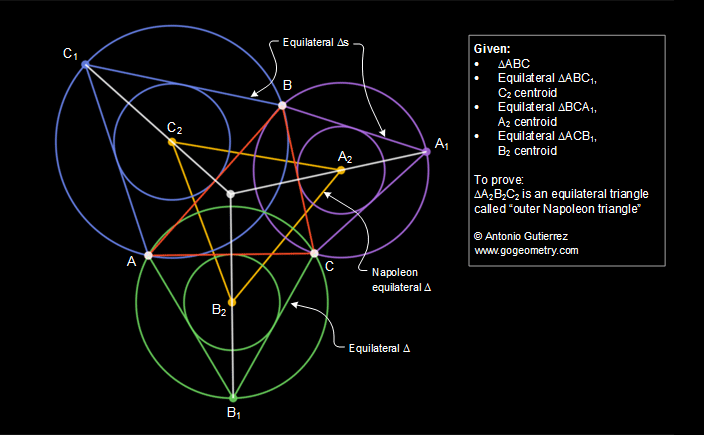# iPad Apps: Apollonius for iPad. Napoleon's Theorem. External Equilateral Triangles. Level: High School, SAT Prep, College, Mathematics Education.

 In the figure below, equilateral triangles ABC1, BCA1, and ACB1 are constructed externally on the sides of a triangle ABC. Prove that the centers A2, B2, and C2, of those equilateral triangles form an equilateral triangle, called the outer Napoleon triangle.See also: Geometry Problem 246. Napoleon's Theorem I.External Equilateral triangles. Outer triangle.